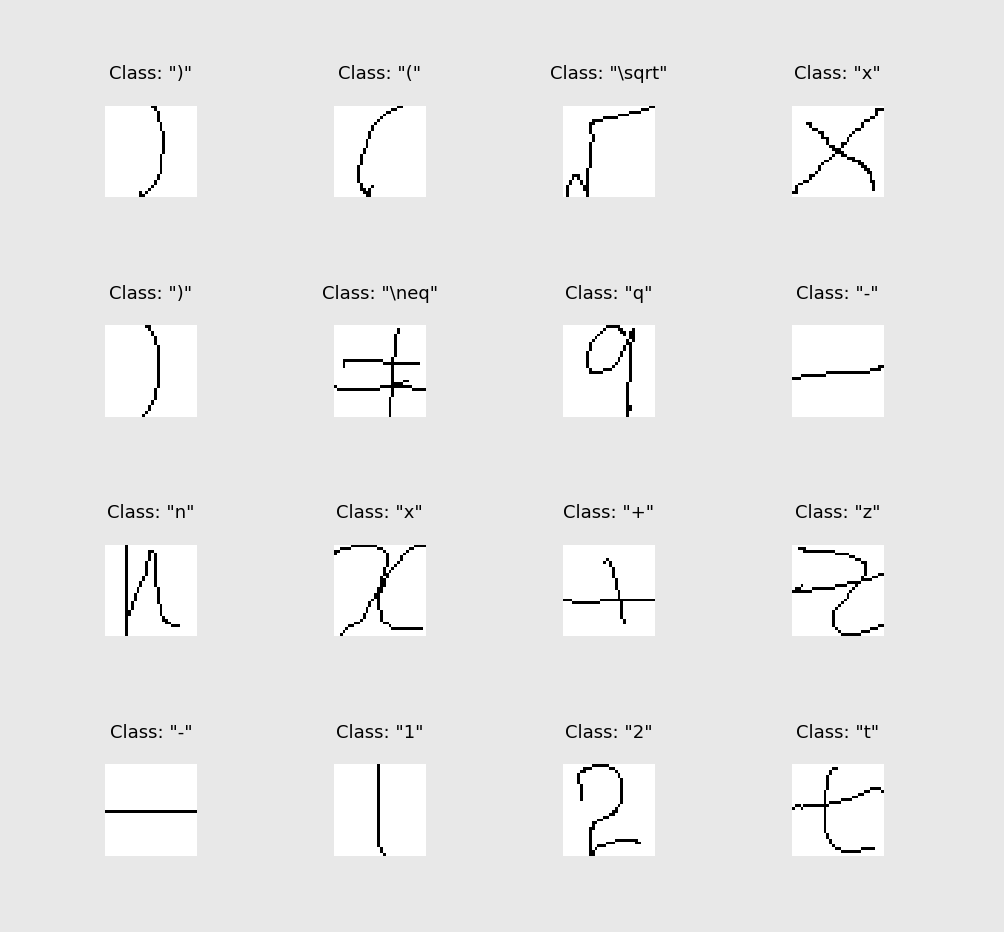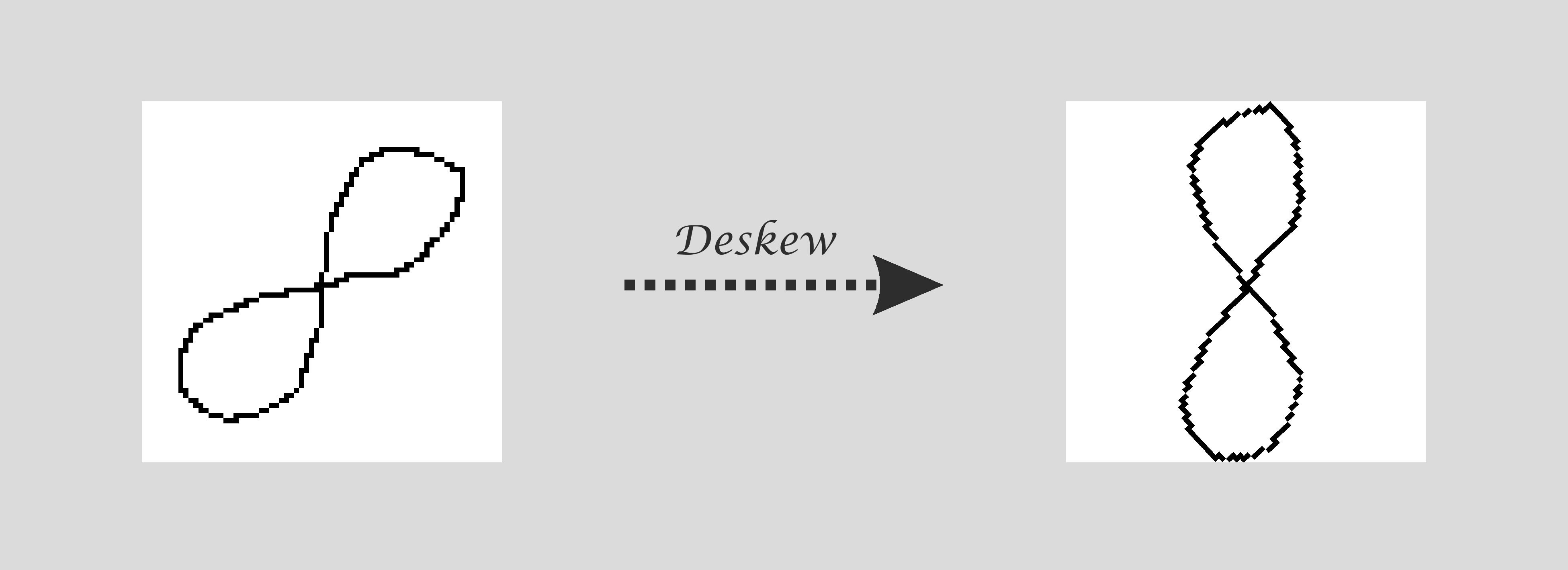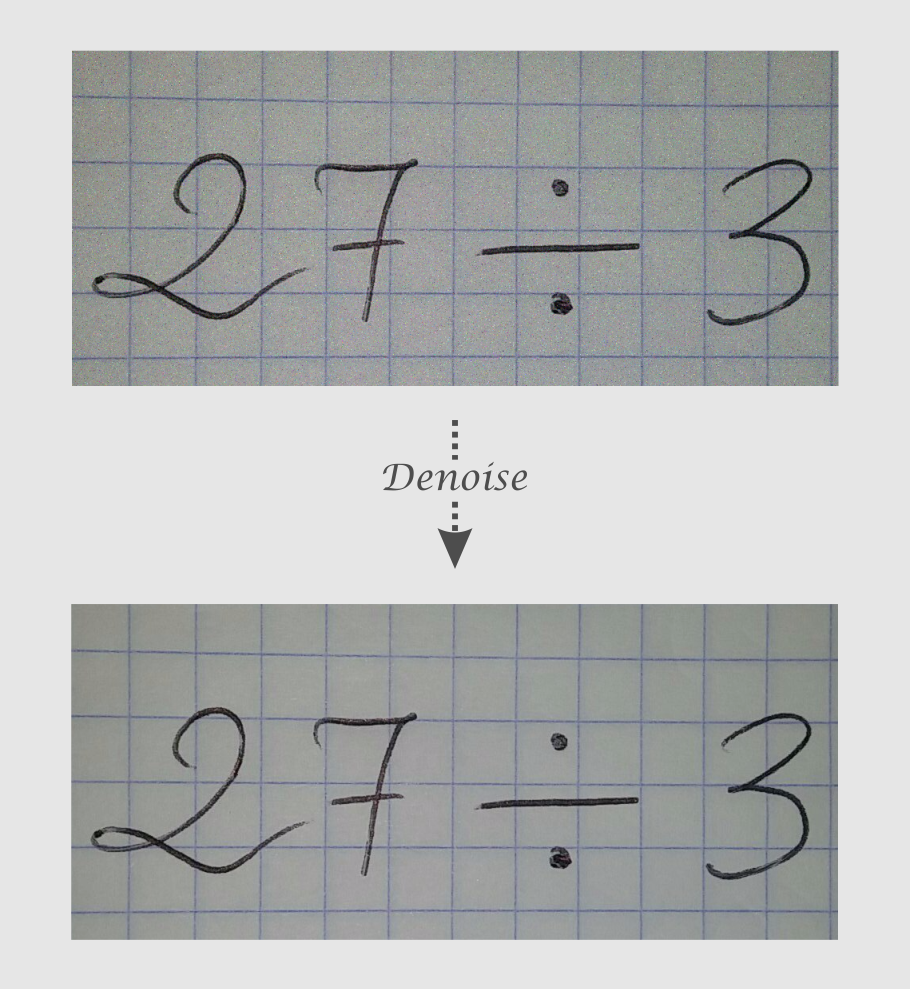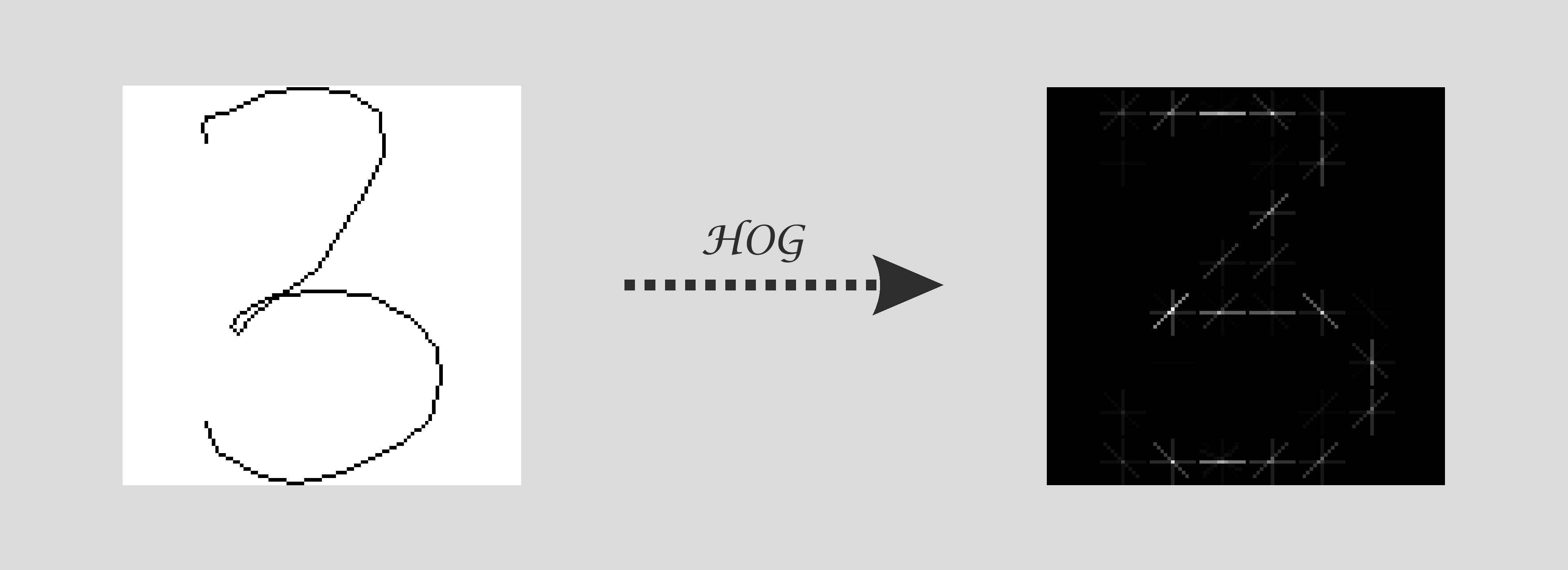After we saved requested image in the database, we want to predict what is on the image and send this prediction back to client.

However, testing/training predictive model on full-sized images would be very unefficient, since there would be infinite number of expression combinations we would have to take care of. What’s more, the dataset wel’ll be training our classifier (predictive model) on, consists of square images, each of which represent a single digit/arithmetic operator.

Let’s acually visualize a few records of our training set:You can notice a few common traits that all records share:

• Images are of the same size.
• Images are binary - they have only two possible values for each pixel (in our case, pixel represents either black or white color).
• Patterns are centered inside square images.
• Patterns were thinned - they are 1 pixel thick.

These are results of data preprocessing. We apply preprocessing techniques in order to standardize the data (introduce a common data representation standard). We do so, because we don’t want our classifier to take the size of a pattern or its thickness into consideration, but rather focus on truly relevant features like pattern strokes themselves.

There are a few other preprocessing techniques that are widely used:

• Image deskewing.

Since our classifier cannot distinguish a crooked image from the straight one, it would have a hard time classifying correct class or it wouldn’t classify it whatsoever. This is where, image deskewing comes in:Prior to straightening an image, we need to compute the skew angle. It turns out, that the most accurate method to compute it is Probabilistic Hough Transform.

• Image noise removal.

Image noise is random (not present in the object imaged) variation of brightness or color information in images, and is usually an aspect of electronic noise.• Better data representation.

In this post, we consider pixel intensities as a feature representation for the symbol images. However, there are numerous other feature representations:

• Histogram of oriented gradients (HOG).

The idea behind HOG feature is that an image can be represented by localized distribution of intensity gradients. That’s why HOG features might appear well suited to text recognition task, as the intensity gradients in an image will be due to symbol strokes themselves.

Visualization of extracted HOG features:• Contour of a pattern.

Contours can be explained simply as a curve joining all the continuous points (along the boundary), having same color or intensity. Storing coordinates of these points in a single array is how you represent a contour in practise.

While describing a pattern in terms of its contour is simpler than its oriented gradients, it is definitely advantageous over pixel intensities representation.

• Limit number of classes.

The more correlated classes our classifier has to learn, the less confident it is in predicting one of those. Hence, you want to algorithmically resolve as many classes as possible.

Let’s take “-“ class into consideration. It stands out among other classes, because of its pattern’s disproportionate size. Based on this fact, we could resolve all the patterns that are dispropotionate in width with respect to height.

Knowing what processing techniques were applied to the records of our training set, we can get down to processing requested images.

### Process request images

Patterns appearing in the requested image have to be converted to the same exact format as the records of our training set.

Before we start to write any code, we need to:

• Downlaod a few dependencies:

• Install them via pip:

``````pip install "scikit_image-0.13.0-cp35-cp35m-win_amd64.whl"
pip install "scipy-0.19.1-cp35-cp35m-win_amd64.whl"
pip install "numpy-1.13.1+mkl-cp35-cp35m-win_amd64.whl"
``````

Yet we need opencv which is available through the Python Package Index:

``````pip install opencv-python
``````

Now, we are ready to code :)

Create a new python file inside your Django project directory and name it image.py as I did.

At the top of newly created file add the following imports:

``````# image.py

from skimage import img_as_ubyte
from skimage.filters import gaussian, threshold_minimum
from skimage.morphology import square, erosion, thin

import numpy as np
import cv2

# ...
``````

I break down the processing algorithm into 2 parts:

• Binarize an image:

``````# image.py

# dependencies ...

# Process requested image
def binarize(image_abs_path):

# Convert color image (3-channel deep) into grayscale (1-channel deep)
# We reduce image dimensionality in order to remove unrelevant features like color.

# Apply Gaussian Blur effect - this removes image noise
gaussian_blur = gaussian(grayscale_img, sigma=1)

# Apply minimum threshold
thresh_sauvola = threshold_minimum(gaussian_blur)

# Convert thresh_sauvola array values to either 1 or 0 (white or black)
binary_img = gaussian_blur > thresh_sauvola

return binary_img

``````
• Extract patterns:

``````# image.py

# dependencies ...

# def process(image_abs_path) ...

def shift(contour):

# Get minimal X and Y coordinates
x_min, y_min = contour.min(axis=0)

# Subtract (x_min, y_min) from every contour point
return np.subtract(contour, [x_min, y_min])

def get_scale(cont_width, cont_height, box_size):

ratio = cont_width / cont_height

if ratio < 1.0:
return box_size / cont_height
else:
return box_size / cont_width

def extract_patterns(image_abs_path):

max_intensity = 1
# Here we define the size of the square box that will contain a single pattern
box_size = 32

binary_img = binarize(image_abs_path)

# Apply erosion step - make patterns thicker
eroded_img = erosion(binary_img, selem=square(3))

# Inverse colors: black --> white | white --> black
binary_inv_img = max_intensity - eroded_img

# Apply thinning algorithm
thinned_img = thin(binary_inv_img)

# Before we apply opencv method, we need to convert scikit image to opencv image
thinned_img_cv = img_as_ubyte(thinned_img)

# Find contours
_, contours, _ = cv2.findContours(thinned_img_cv, mode=cv2.RETR_EXTERNAL, method=cv2.CHAIN_APPROX_SIMPLE)

# Sort contours from left to right (sort by bounding rectangle's X coordinate)
contours = sorted(contours, key=lambda cont: cv2.boundingRect(cont))

# Initialize patterns array
patterns = []

for contour in contours:

# Initialize blank white box that will contain a single pattern
pattern = np.ones(shape=(box_size, box_size), dtype=np.uint8) * 255

# Shift contour coordinates so that they are now relative to its square image
shifted_cont = shift(contour)

# Get size of the contour
cont_width, cont_height = cv2.boundingRect(contour)[2:]
# boundingRect method returns width and height values that are too big by 1 pixel
cont_width -= 1
cont_height -= 1

# Get scale - we will use this scale to interpolate contour so that it fits into
# box_size X box_size square box.
scale = get_scale(cont_width, cont_height, box_size)

# Interpolate contour and round coordinate values to int type
rescaled_cont = np.floor(shifted_cont * scale).astype(dtype=np.int32)

# Get size of the rescaled contour
rescaled_cont_width, rescaled_cont_height = cont_width * scale, cont_height * scale

# Get margin
margin_x = int((box_size - rescaled_cont_width) / 2)
margin_y = int((box_size - rescaled_cont_height) / 2)

# Center pattern wihin a square box - we move pattern right by a proper margin

# Draw centered contour on a blank square box
cv2.drawContours(pattern, [centered_cont], contourIdx=0, color=(0))

patterns.append(pattern)

return patterns
``````

Every time someone sends an image to our server, we want to extract patterns and feed these patterns into a classifier.

``````# digits_operators_recognizer/resolver/views.py

# dependencies ...
from ocr import image

# ...

class ImageCreate(CreateAPIView):

'Create a new image instance'

serializer_class = ImageSerializer

def post(self, request):

serializer = ImageSerializer(data=request.data)
if serializer.is_valid():

# Save request image in the database
serializer.save()

# We need to remove slash from the beginning of the path string
image_path = serializer.data.get('image')[1:]
image_abs_path = os.path.join(settings.BASE_DIR, image_path)

# Extract patterns
patterns = image.extract_patterns(image_abs_path)

return Response(serializer.data, status=status.HTTP_201_CREATED)